# Plane Geometry Review : Triangle Area calculation

Area Formulas

1.  Given base and altitudeTriangle: Given base and height
Area = 1/2 (b)(h)

2. Given two sides and an AngleTriangle:  Given 2 sides and angle
Area = 1/2 (a) (b)sin θ

3. Given 3 sidesTriangle Given 3 sides a,b,c

Area = sqrt (s(s-a)(s-b)(s-c))----->  Heron's Formula
where s= (a+b+c)/2

4. Triangle inscribed in a circler= radius of the circle
Area = (a b c)/ 4r

5. Given a circle inscribed in a Triangle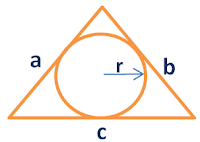r = radius of the circle

Area  =  rs
s = ( a+b+c/) 2

6. Circle escribed by the triangle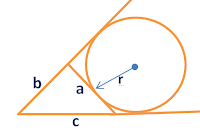where a is the side tangent to the circle
Area =  r (s-a)
s = ( a+b+c/) 2

# Engineering Mathematics : Plane Geometry Part 1

Fundamental Principles

Angles

• Acute angle = less 90 deg
• Obtuse angle = more than 90 and less than 180 deg
• Straight angle =180 deg
• Vertex of the angle is point where two lines meet
• Supplementary angles have a sum of 180 deg
• Complementary angles have a sum of 90 deg
• The base angles of an isosceles (two equal sides) triangle =  60 deg
• Each angle of an isosceles right triangle = 45 deg
• Exterior angle of a triangle is the sum of the remote interior angle
• The sum of the angles of a polygon of n sides = (n-2)180 deg
• The sum of the exterior angles of any polygon = 360 deg

Congruence of triangle
In geometry,Two triangles are congruent if their corresponding sides are equal in length and their corresponding angles are equal in size

conditions:   (s=side  ; a=angle)
• s.a.s =s.a.s   (Side-Angle-Side)
• a.s.a=a.s.a  (Angle-Side-Angle)
• s.s.s=s.s.s  (Side-Side-Side
• a.a.s=a.a.s (Angle-Angle-Side)

Two right triangles are congruent if hypotenuse and leg of one hypotenuse and corresponding leg of the other.

# Algebra: What are the examples of Empirical formula

Some Formulas are derived by means of experimentation. Among these are the formulas discovered by pascal, Galileo and Archimedes. They are called empirical formulas.

Examples of Empirical formulas:
1.1 a formula for changing temperature from Fahrenheit to centigrade.
2.   a formula showing the relation of weights on a balance with the lengths of its arms.
3. -a formula used in finding electrical power.
4.a formula for finding work done in foot pounds.
5. an ohms law, a formula for finding I, the number of amperes of electric current , E, the electromotive force in volts, and R, the resistance in ohms.
6. a formula for finding the distance covered by falling bodies.

# Algebra: What is the Lowest common Multiple in Algebraic Expression

The lowest common multiple ( L.C.M) of two or more arithmetical or algebraic expressions is the expression having the least number of factors which  will exactly contain each of the iven expressions.Thus the numbers 4, 6 and 12 will have 12 for their LCM for it exactly contains each of the numbers. it is easier  to find the LCM  of the polynomials like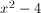and

We find that,  while

Therefore, the LCM of the polynomials is
Note:
To find the LCM of two or more expressions, separate each expression into its prime factors. Then take the product of all the different prime factors using each factor the greatest number of times it occurs in any one expression

# Algebra: How to factor a trinomial Perfect Square

From your previous topics , You learned that the square of a binomial is a trinomial perfect square. Thus , the factors of a trinomial perfect square are two binomials which may be written as the square of a binomial.

To illustrate this point , let us consider the following examples.
Example.1
Multiplication

factoring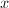is the square root of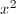while 3 is the square root of 9

Example 2
Multiplication
factoringis the square root ofwhile 5 is the square root of 25

Note:
From the examples , it is clear that the following points should be noted in factoring a trinomial perfect square.
1.The factors consists pf like binomials whose terms are the square roots of the terms which are perfect squares.
2. The sign connecting the terms of the binomial factor is the same as the sign of the remaining term.

Hence if a trinomial is perfect square, its factors can be easily be determined.
The problem then is , " How do we know that a trinomial is a perfect square?"

let us consider the trinomial
Analysis:

therefore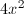and 9 are perfect squares . 2x is called the square root ofand 3 is the square root of 9. Now

and if their product is doubled , we get

which is the middle term of the trinomial.
Therefore, trinomial is a perfect square if the following conditions exist:
1. Two terms are positive perfect squares.
2. The third term (generally the middle term) is twice the product of the square roots of the terms which are perfect and may be either positive or negative.

# Algebra: How to Translate verbal Statements into symbols

An equation is an important tool in problem solving but another tool which is just as necessary is representing related quantities by means of symbols. It must be recalled thatb letter like x can be used to represent the numerical value of a certain quantity. quantities related to the latter may also be expressed in terms of x . For example , if x represents the first of two consecutive integers, then the next consecutive integer( which is greater by 1) will be represented by x+1.

There are certain rules which must be followed in representing quantities. generally, it is sufficient to use x or any chosen symbol to represent the smaller of two quantities or the smallest of three or more quantities. another way is by representing the independent quantity by x. suppose , for example, that Pedro is twice as old as Juan. this condition shows that the age of Pedro depends upon the age of Juan and hence it is better to let x represent the age in years of Juan. Pedro's age in years will re represented by 2x

# Algebra: What is mean Proportion and How to solve it?

A proportion such as 1:2 =2:4 or a:b = b:c  is called a mean proportion because the second and the third terms are equal. In the latter b is the mean proportional between the first and the last terms a and c, respectively and c is the third proportional to a and b.

Solving for the mean proportionalGiven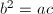in proportion, the product of the extremes is equal to the product of the means.extracting the square roots of booth members of the equation

Note; like roots and like powers of equal numbers are equal

It follows that:
The mean proportional between two quantities is equal to the square root of their product.

example 1 :
Solve for x in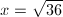example 2 :
Solve for x in

# Algebra: How to solve the Equations containing decimals

Equations containing decimals are equations with fractions. hence the solution of decimal equations is similar to that of fractional equations with this difference-in decimal equation the multiplier of both members is that power
of ten that will clear the equations of its decimal.

Example1.
Solve for x:                                 .3x + 5 = .2x
Multiplying both members
of the equation by 10,                 3x + 50 = 2x
x = -50

Check                                     .3x(-50) + 5 = .2(-50)
-15+5  = -10
-10 = -10

Example no 2.                                      Solve for y in the equation
.5y/3 - .18/4 = .1y/6
Clear the equation of decimals by multiplying both members by 100
50y/3 - 18/4 = 10y/6
Clear the equation of fractions by multiplying both members by 12, the LCD
200y - 54 = 20y
180y = 54
y = 54/180 = .3

check:
.5y/3 - .18/4 = .1y/6
(.5(.3))/3 -.18/4  = (.1(.3))/6
.05-.045 = 0.005
.005 = .005

# Algebra : How to solve a Literal equation

Equations where the known quantities are expressed as letters are called literal equations. Formulas are literal equations. Generally, the last letter of the alphabet are used to represent the unknown quantities, and the first letters, the known quantities.

Examples:

Solve for the unknown and check

1. 3x + 2a = 2x+5a     transposing
3x - 2x = 5a - 2a      combining like terms
x = 3a

check
3.3a + 2a = 2.3a + 5a
9a + 2a = 6a + 5a
11a = 11a

2. 8z + 6m = 5z + 9m
8z - 5z = 9m - 6m        transposing
3z = 3m                   combining like terms
z = m                       axiom of division

check
8.m + 6m = 5.m + 9.m
8m + 6m= 5m + 9m
14m = 14m